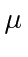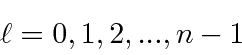# Hydrogen

The Hydrogen atom consists of an electron bound to a proton by the Coulomb potential.We can generalize the potential to a nucleus of chargewithout complication of the problem.Since the potential is spherically symmetric, the problem separates and the solutions will be a product of a radial wavefunction and one of the spherical harmonics.We have already studied the spherical harmonics.

The radial wavefunction satisfies the differential equation that depends on the angular momentum quantum number,whereis the reduced mass of the nucleus and electron.The differential equation can be solved using techniques similar to those used to solve the 1D harmonic oscillator equation. We find the eigen-energiesand the radial wavefunctionswhere the coefficients of the polynomials can be found from the recursion relationandThe principle quantum numberis an integer from 1 to infinity.This principle quantum number is actually the sum of the radial quantum number plusplus 1.and therefore, the total angular momentum quantum numbermust be less than.This unusual way of labeling the states comes about because a radial excitation has the same energy as an angular excitation for Hydrogen. This is often referred to as an accidental degeneracy.

Subsections
Jim Branson 2013-04-22